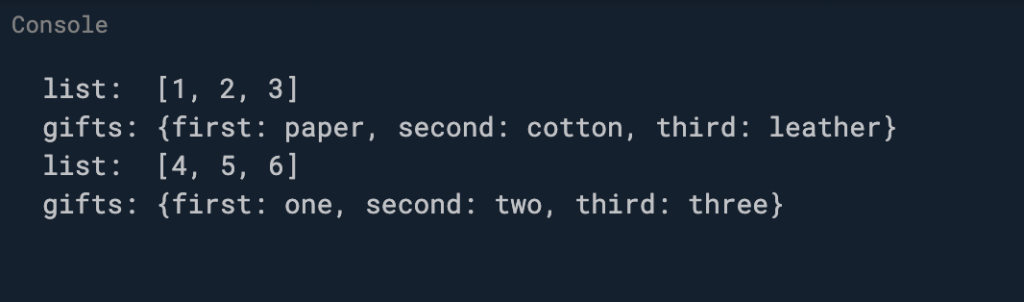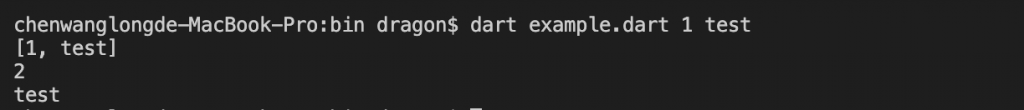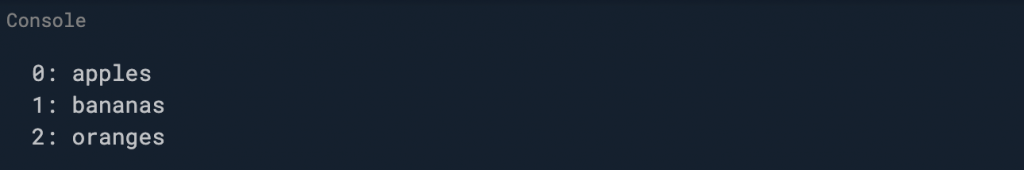0

# Functions 函數(方法)

## 定義

``````//定義一個函數 判斷清單對應參數是否為null
bool isNoble(int atomicNumber) {
return list[atomicNumber] != null;
}
``````

``````//定義一個函數 判斷清單對應參數是否為null 縮寫寫法
bool isNoble(int atomicNumber) => list[atomicNumber] != null;
``````

=> 胖箭頭: 簡寫語法用於僅包含一句表達式的函數。這個語法特別是在將匿名函數作為參數傳遞時非常有用。

## 參數

### 命名參數

``````void enableFlags({bool bold, bool hidden}) {...}
``````

``````enableFlags(bold: true, hidden: false);
``````

``````const Scrollbar({Key key, @required Widget child})
``````

@required 註解定義在 meta 套件中，可以直接導入 package:meta/meta.dart 使用。

### 位置参数

``````String say(String from, String msg, [String device]) {
var result = '\$from says \$msg';
if (device != null) {
result = '\$result with a \$device';
}
return result;
}
``````

``````assert(say('Bob', 'Howdy') == 'Bob says Howdy');
``````

``````assert(say('Bob', 'Howdy', 'smoke signal') == 'Bob says Howdy with a smoke signal');
``````

### 預設參數值

``````void main() {
String say(String from, String msg,
[String device = 'carrier pigeon', String mood]) {
var result = '\$from says \$msg';
if (device != null) {
result = '\$result with a \$device';
}
if (mood != null) {
result = '\$result (in a \$mood mood)';
}
return result;
}

assert(say('Bob', 'Howdy') == 'Bob says Howdy with a carrier pigeon');
}
``````

List 或 Map 同樣也可以作為預設值。下面的範例定義了一個 doStuff() 的函數，其中的參數 list 和 gifts 指定了一個 List 類型的值和 Map 類型的值。

``````void main() {
var list1 = [4,5,6];
var map1 = {'first':'one','second':'two','third':'three'};

void doStuff(
{List<int> list = const [1, 2, 3],
Map<String, String> gifts = const {
'first': 'paper',
'second': 'cotton',
'third': 'leather'
}}) {
print('list:  \$list');
}
doStuff();
}
````````````//num a, num b, num c, num d 最普通的傳參: 呼叫時，引數個數和引數順序必須固定
add1(num a, num b, num c, num d) {
print(a + b + c + d);
}

//[num a, num b, num c, num d]傳參: 呼叫時，引數個數不固定，但是引數順序需要一一對應, 不支援命名引數
add2([num a, num b, num c, num d]) {
print(a + b + c + d);
}

//{num a, num b, num c, num d}傳參: 呼叫時，引數個數不固定，引數順序也可以不固定，支援命名引數,也叫可選引數，是dart中的一大特性，這就是為啥Flutter程式碼那麼多可選屬性，大量使用可選引數
add3({num a, num b, num c, num d}) {
print(a + b + c + d);
}

//num a, num b, {num c, num d}傳參: 呼叫時，a,b引數個數固定順序固定，c,d引數個數和順序也可以不固定
add4(num a, num b, {num c, num d}) {
print(a + b + c + d);
}

main() {
add1(100, 100, 100, 100); //最普通的傳參: 呼叫時，引數個數和引數順序必須固定
b: 200,
a: 200,
c: 100,
d: 100); //呼叫時，引數個數不固定，引數順序也可以不固定，支援命名引數(也就意味著順序可變)
add4(100, 100, d: 100, c: 100); //呼叫時，a,b引數個數固定順序篤定，c,d引數個數和順序也可以不固定
}
``````
``````add3({num a, num b, num c, num d = 100}) {//d就是預設值引數，給的預設值是100
print(a + b + c + d);             //1200
}

main() {
add3(b: 200, a: 100, c: 800);
}
``````
``````main() {
Function square = (a) {
return a * a;
};

Function square2 = (a) {
return a * a * a;
};
}

num add(num a, num b, [Function op, Function op2]) {
//函式作為引數傳遞
return op(a) + op2(b);
}
``````

## main() 函數

``````void main(List<String> arguments) {
print(arguments);
print(arguments.length);
print(arguments);
}
``````## 匿名函數

([[類型] 參數[, …]]) {

};

``````void main() {
var loudify = (msg) => '!!! \${msg.toUpperCase()} !!!';
print(loudify('hello'));            //!!! HELLO !!!
}
``````
``````void main() {
var list = ['apples', 'bananas', 'oranges'];
list.forEach((item) {
print('\${list.indexOf(item)}: \$item');
});
}
``````## 支援閉包(closure)

``````Function makeAdder(int addBy) {
return (int i) => addBy + i;
}

void main() {
// 生成加 2 的函数。